# Relativistic electromagnetism

Static magnetic field

Edward M. Purcell Electricity and Magnetism, Berkeley Physics Course, Vol. 2 in SI units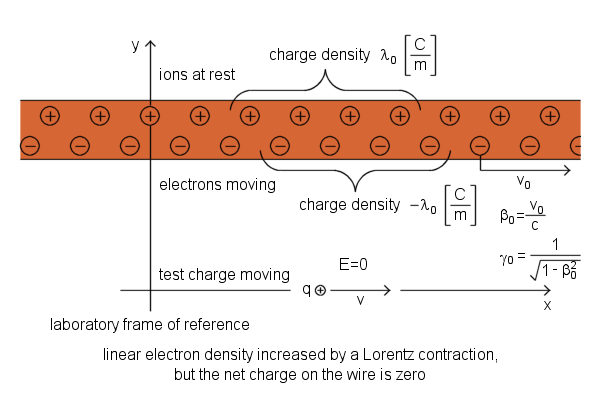Current-Carrying Wire, positive ions and electrons are shown separated for clarity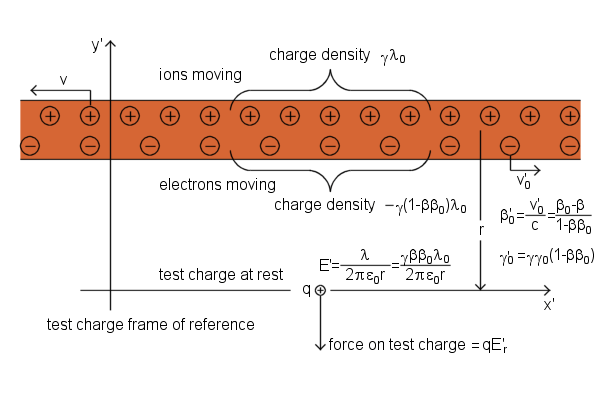Current-Carrying Wire, positive ions and electrons are shown separated for clarity

The linear density of positive charge in the test charge frame is
γλ0
Calculation of the linear density of negative charge in the test charge frame:
The electron linear density in the lab frame, which was - λ0, had already been increased by a Lorentz contraction.
Thus the linear density of negative charge in the electrons' own rest frame must have been
- λ00
Now we calculate the speed of the electrons in the test charge frame in order to calculate their density there:
Relativistic formula for the addition of velocities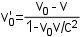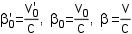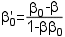Lorentz factor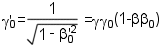The total linear density of charge in the wire in the test charge frame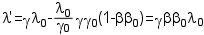A radial electric field of infinite line charge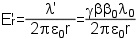Force on test charge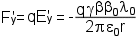For the observer in the test charge frame wire is length contracted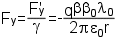The total current I in the wire
- λ0v0 or - λ0β0c
Force on test charge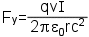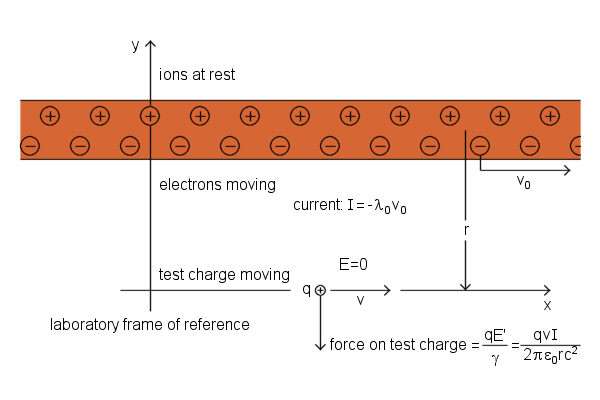Current-Carrying Wire, positive ions and electrons are shown separated for clarity

Interaction between a current-carrying wire and charge moving at right angle to the wire.

Edward M. Purcell Electricity and Magnetism, Berkeley Physics Course, Vol. 2 in SI units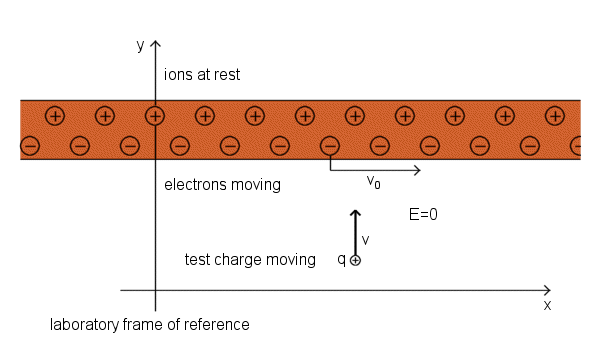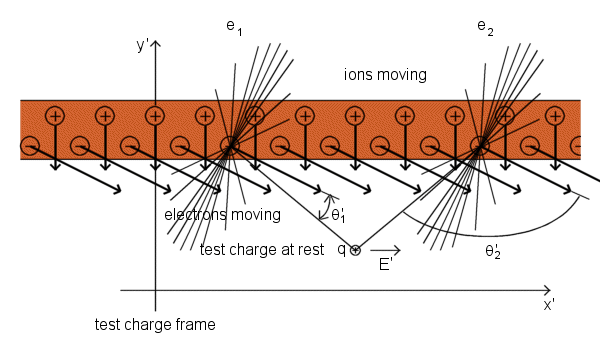Charge moving perpendicular to the wire experiences a force parallel to the wire, perpendicular to its direction of motion. Positive ions cannot cause a horizontal field at the test charge position. The x' component of the field from an ion on the left is exactly cancelled by the x' component of the field of a symmetrically positioned ion on the right. The effect we can see is caused by the electrons. Electrons are all moving diagonally in the test charge frame, downward and toward the right. Consider the two symmetrically located electrons e1 and e2. Their electric fields, relativistically compressed in the direction of the electrons' motion, are represented by a field lines. You can see that, although e1 and e2 are equally far away from the test charge, the field of electron e2 is stronger than the field of electron e1 at that location. That is because the line from e2 to the test charge is more nearly perpendicular to the direction of motion of e2. In other words, the angle θ' that appears in the denominator of equation for the magnitude of the field of electron in the frame moving relative to the electron is here different for e1 and e2, so that sin2θ'2 > sin2θ'1. That is true for any symmetrically located pair of electrons on the line, as you can verify with the aid of picture. The electron on the right always acts stronger. Therefore, summing over all the electrons must give a resultant field E' in the x direction. The y' component of the electrons' field is exactly cancelled by the field of the ions. That E'y is zero is guaranteed by Gauss's law, for the number of charges per unit length of wire is the same as it was in the lab frame. The wire is uncharged in both frames. The force on the test charge, qE'x, transformed back into the lab frame is a force proportional to v in the x direction, which is the direction of v×B if B is a vector in the z direction, pointing out of the page.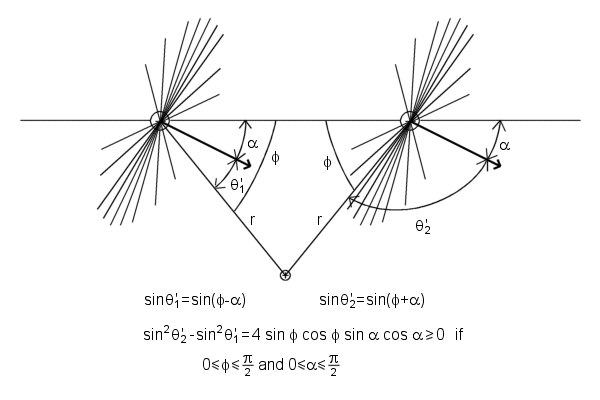relativistic, electromagnetism, magnetic, field, electromagnetic, induction, physics, science,